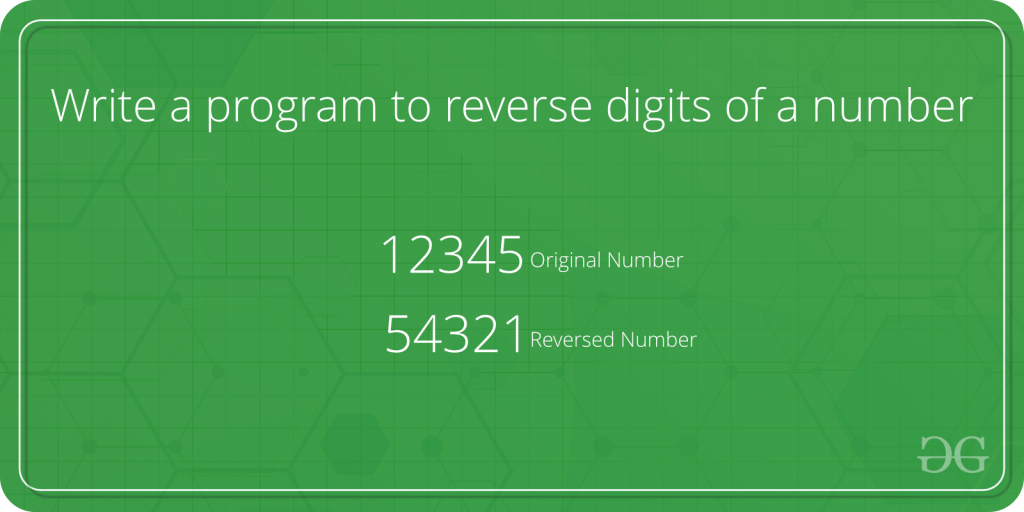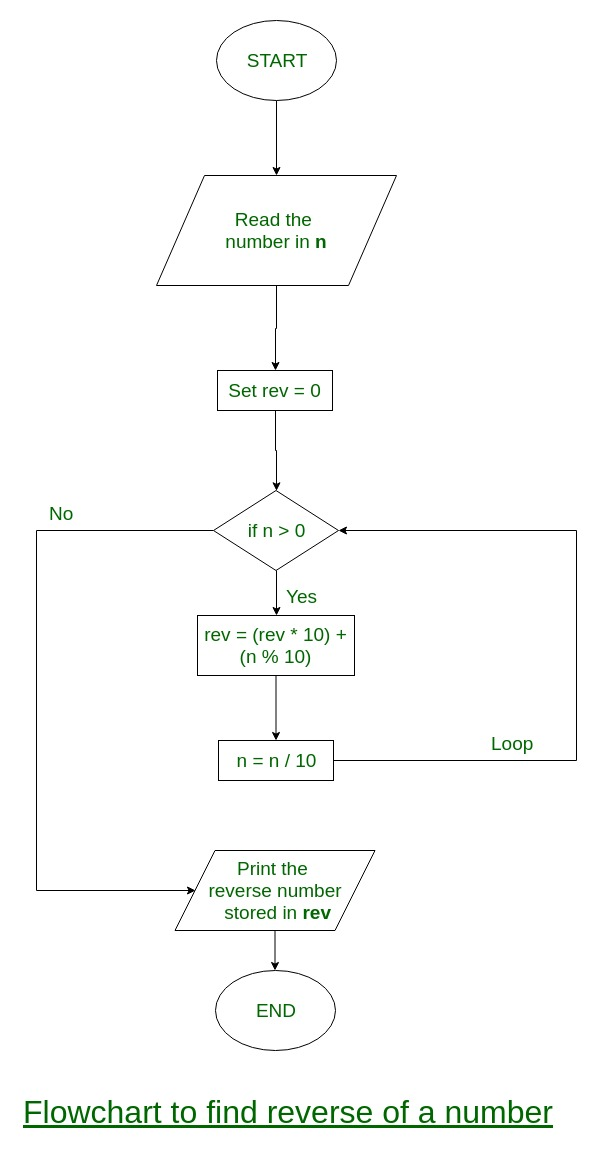# Write a program to reverse digits of a number

Write a program to reverse digits of an integer.Examples :

```Input : num = 12345
Output : 54321

Input : num = 876
Output : 678
```

## Recommended: Please solve it on “PRACTICE ” first, before moving on to the solution.

Flowchart:ITERATIVE WAY
Algorithm:

```Input:  num
(1) Initialize rev_num = 0
(2) Loop while num > 0
(a) Multiply rev_num by 10 and add remainder of num
divide by 10 to rev_num
rev_num = rev_num*10 + num%10;
(b) Divide num by 10
(3) Return rev_num
```

Example:
num = 4562
rev_num = 0

rev_num = rev_num *10 + num%10 = 2
num = num/10 = 456

rev_num = rev_num *10 + num%10 = 20 + 6 = 26
num = num/10 = 45

rev_num = rev_num *10 + num%10 = 260 + 5 = 265
num = num/10 = 4

rev_num = rev_num *10 + num%10 = 265 + 4 = 2654
num = num/10 = 0

Program:

## C++

 `#include ` ` `  `using` `namespace` `std; ` `/* Iterative function to reverse digits of num*/` `int` `reversDigits(``int` `num) ` `{ ` `    ``int` `rev_num = 0; ` `    ``while``(num > 0) ` `    ``{ ` `        ``rev_num = rev_num*10 + num%10; ` `        ``num = num/10; ` `    ``} ` `    ``return` `rev_num; ` `} ` ` `  `/*Driver program to test reversDigits*/` `int` `main() ` `{ ` `    ``int` `num = 4562; ` `    ``cout << ``"Reverse of no. is "` `         ``<< reversDigits(num); ` `    ``getchar``(); ` `    ``return` `0; ` `} ` ` `  `// This code is contributed ` `// by Akanksha Rai(Abby_akku) `

## C

 `#include ` ` `  `/* Iterative function to reverse digits of num*/` `int` `reversDigits(``int` `num) ` `{ ` `    ``int` `rev_num = 0; ` `    ``while``(num > 0) ` `    ``{ ` `        ``rev_num = rev_num*10 + num%10; ` `        ``num = num/10; ` `    ``} ` `    ``return` `rev_num; ` `} ` ` `  `/*Driver program to test reversDigits*/` `int` `main() ` `{ ` `    ``int` `num = 4562; ` `    ``printf``(``"Reverse of no. is %d"``, reversDigits(num)); ` ` `  `    ``getchar``(); ` `    ``return` `0; ` `} `

## Java

 `// Java program to reverse a number  ` ` `  `class` `GFG ` `{ ` `    ``/* Iterative function to reverse ` `    ``digits of num*/` `    ``static` `int` `reversDigits(``int` `num) ` `    ``{ ` `        ``int` `rev_num = ``0``; ` `        ``while``(num > ``0``) ` `        ``{ ` `            ``rev_num = rev_num * ``10` `+ num % ``10``; ` `            ``num = num / ``10``; ` `        ``} ` `        ``return` `rev_num; ` `    ``} ` `     `  `    ``// Driver code ` `    ``public` `static` `void` `main (String[] args)  ` `    ``{ ` `        ``int` `num = ``4562``; ` `        ``System.out.println(``"Reverse of no. is "`  `                           ``+ reversDigits(num)); ` `    ``} ` `} ` ` `  `// This code is contributed by Anant Agarwal. `

## Python

 `# Python program to reverse a number  ` ` `  `n ``=` `4562``; ` `rev ``=` `0` ` `  `while``(n > ``0``): ` `    ``a ``=` `n ``%` `10` `    ``rev ``=` `rev ``*` `10` `+` `a ` `    ``n ``=` `n ``/``/` `10` `     `  `print``(rev) ` ` `  `# This code is contributed by Shariq Raza  `

## C#

 `// C# program to reverse a number  ` `using` `System; ` ` `  `class` `GFG ` `{ ` `    ``// Iterative function to  ` `    ``// reverse digits of num ` `    ``static` `int` `reversDigits(``int` `num) ` `    ``{ ` `        ``int` `rev_num = 0; ` `        ``while``(num > 0) ` `        ``{ ` `            ``rev_num = rev_num * 10 + num % 10; ` `            ``num = num / 10; ` `        ``} ` `        ``return` `rev_num; ` `    ``} ` `     `  `    ``// Driver code ` `    ``public` `static` `void` `Main()  ` `    ``{ ` `        ``int` `num = 4562; ` `        ``Console.Write(``"Reverse of no. is "` `                        ``+ reversDigits(num)); ` `    ``} ` `} ` ` `  `// This code is contributed by Sam007 `

## PHP

 ` 1) ` `    ``{ ` `        ``\$rev_num` `= ``\$rev_num` `* 10 +  ` `                        ``\$num` `% 10; ` `        ``\$num` `= (int)``\$num` `/ 10; ` `    ``} ` `    ``return` `\$rev_num``; ` `} ` ` `  `// Driver Code ` `\$num` `= 4562; ` `echo` `"Reverse of no. is "``,  ` `       ``reversDigits(``\$num``); ` ` `  `// This code is contributed by aj_36 ` `?> `

Time Complexity: O(Log(n)) where n is the input number.
Output:

```2654
```

RECURSIVE WAY
Thanks to Raj for adding this to the original post.

## C++

 `// C++ program to reverse digits of a number ` `#include ` `using` `namespace` `std; ` `/* Recursive function to reverse digits of num*/` `int` `reversDigits(``int` `num) ` `{ ` `static` `int` `rev_num = 0; ` `static` `int` `base_pos = 1; ` `if``(num > 0) ` `{ ` `    ``reversDigits(num/10); ` `    ``rev_num += (num%10)*base_pos; ` `    ``base_pos *= 10; ` `} ` `return` `rev_num; ` `} ` ` `  `// Driver Code ` `int` `main() ` `{ ` `    ``int` `num = 4562; ` `    ``cout << ``"Reverse of no. is "`  `         ``<< reversDigits(num); ` ` `  `    ``return` `0; ` `} ` ` `  `// This code is contributed  ` `// by Akanksha Rai(Abby_akku) `

## C

 `// C program to reverse digits of a number ` `#include ; ` ` `  `/* Recursive function to reverse digits of num*/` `int` `reversDigits(``int` `num) ` `{ ` `  ``static` `int` `rev_num = 0; ` `  ``static` `int` `base_pos = 1; ` `  ``if``(num > 0) ` `  ``{ ` `    ``reversDigits(num/10); ` `    ``rev_num  += (num%10)*base_pos; ` `    ``base_pos *= 10; ` `  ``} ` `  ``return` `rev_num; ` `} ` ` `  `/*Driver program to test reversDigits*/` `int` `main() ` `{ ` `    ``int` `num = 4562; ` `    ``printf``(``"Reverse of no. is %d"``, reversDigits(num)); ` ` `  `    ``getchar``(); ` `    ``return` `0; ` `} `

## Java

 `// Java program to reverse digits of a number ` ` `  `// Recursive function to  ` `// reverse digits of num ` `class` `GFG ` `{ ` `static` `int` `rev_num = ``0``; ` `static` `int` `base_pos = ``1``; ` `static` `int` `reversDigits(``int` `num) ` `{ ` `    ``if``(num > ``0``) ` `    ``{ ` `        ``reversDigits(num / ``10``); ` `        ``rev_num += (num % ``10``) * base_pos; ` `        ``base_pos *= ``10``; ` `    ``} ` `return` `rev_num; ` `} ` ` `  `// Driver Code ` `public` `static` `void` `main(String[] args) ` `{ ` `    ``int` `num = ``4562``; ` `    ``System.out.println(reversDigits(num)); ` `} ` `} ` ` `  `// This code is contributed by mits `

## Python3

 `# Python 3 program to reverse digits ` `# of a number ` `rev_num ``=` `0` `base_pos ``=` `1` ` `  `# Recursive function to reverse ` `# digits of num ` `def` `reversDigits(num): ` `    ``global` `rev_num ` `    ``global` `base_pos ` `    ``if``(num > ``0``): ` `        ``reversDigits((``int``)(num ``/` `10``)) ` `        ``rev_num ``+``=` `(num ``%` `10``) ``*` `base_pos ` `        ``base_pos ``*``=` `10` `    ``return` `rev_num ` `     `  `# Driver Code  ` `num ``=` `4562` `print``(``"Reverse of no. is "``, ` `         ``reversDigits(num)) ` `     `  `# This code is contributed by Rajput-Ji `

## C#

 `// C# program to reverse digits of a number ` ` `  `// Recursive function to  ` `// reverse digits of num ` `using` `System; ` `class` `GFG ` `{ ` `static` `int` `rev_num = 0; ` `static` `int` `base_pos = 1; ` `static` `int` `reversDigits(``int` `num) ` `{ ` `    ``if``(num > 0) ` `    ``{ ` `        ``reversDigits(num / 10); ` `        ``rev_num += (num % 10) * base_pos; ` `        ``base_pos *= 10; ` `    ``} ` `return` `rev_num; ` `} ` ` `  `// Driver Code ` `public` `static` `void` `Main() ` `{ ` `    ``int` `num = 4562; ` `    ``Console.WriteLine(reversDigits(num)); ` `} ` `} ` ` `  `// This code is contributed  ` `// by inder_verma `

## PHP

 ` 0)  ` `    ``{  ` `        ``reversDigits((int)(``\$num` `/ 10));  ` `        ``\$rev_num` `+= (``\$num` `% 10) *  ` `                     ``\$base_pos``;  ` `        ``\$base_pos` `*= 10;  ` `    ``}  ` `    ``return` `\$rev_num``;  ` `}  ` ` `  `// Driver Code ` `\$num` `= 4562;  ` `echo` `"Reverse of no. is "``,  ` `       ``reversDigits(``\$num``);  ` ` `  `// This code is contributed by ajit ` `?> `

Output:

```Reverse of no. is 2654
```

Time Complexity: O(Log(n)) where n is the input number.

Reverse digits of an integer with overflow handled

Note that above above program doesn’t consider leading zeroes. For example, for 100 program will print 1. If you want to print 001 then see this comment from Maheshwar.

Try extensions of above functions that should also work for floating point numbers.

Attention reader! Don’t stop learning now. Get hold of all the important DSA concepts with the DSA Self Paced Course at a student-friendly price and become industry ready.

My Personal Notes arrow_drop_up

Article Tags :

11

Please write to us at contribute@geeksforgeeks.org to report any issue with the above content.